# Test Prep Plan - Take a practice test

Take this practice test to check your existing knowledge of the course material. We'll review your answers and create a Test Prep Plan for you based on your results.
How Test Prep Plans work
1
2Based on your results, we'll create a customized Test Prep Plan just for you!
3Study smarter
Study more effectively: skip concepts you already know and focus on what you still need to learn.

# Calculating Derivatives Chapter Exam

Exam Instructions:

Choose your answers to the questions and click 'Next' to see the next set of questions. You can skip questions if you would like and come back to them later with the yellow "Go To First Skipped Question" button. When you have completed the practice exam, a green submit button will appear. Click it to see your results. Good luck!

### Page 1

#### Question 1 1. Which of the following statements is true about the derivative of f(x)?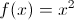#### Question 2 2. What is the derivative of f(z)?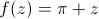#### Question 3 3. Find the jerk of the function shown below: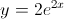#### Question 4 4. What is the highest-order derivative of f(x) that is NOT zero?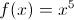#### Question 5 5. Find the second derivative of f(x) shown below: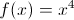### Page 2

#### Question 6 6. The graph x(t) represents the position of an object as a function of time. What is the acceleration of the object for t>0?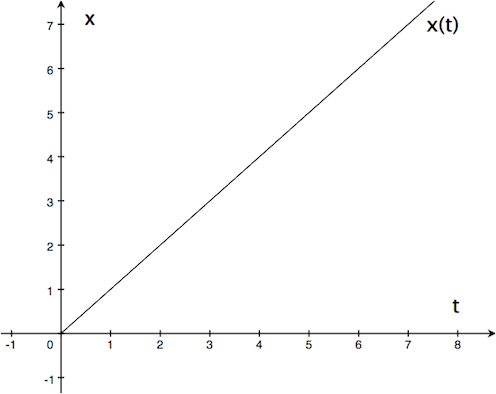#### Question 7 7. Given the derivative of a function (ƒ′(x)), which of the following could be ƒ(x)?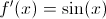#### Question 8 8. Calculate the derivative of f(x), and evaluate it at x=2.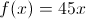#### Question 9 9. Find the derivative of f(x).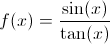#### Question 10 10. Find the derivative of f(x).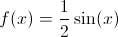### Page 3

#### Question 11 11. Evaluate the following second order derivative.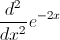#### Question 12 12. What is the derivative of f(x)?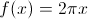#### Question 13 13. What is the derivative of f(x)?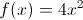#### Question 14 14. Which of the following statements is true, given g(t) below?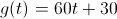#### Question 15 15. What is the derivative of y?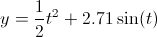### Page 4

#### Question 16 16. Calculate the derivative of g(t).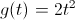#### Question 17 17. If the following graph is f(x), then which of the graphs below could be f''(x)?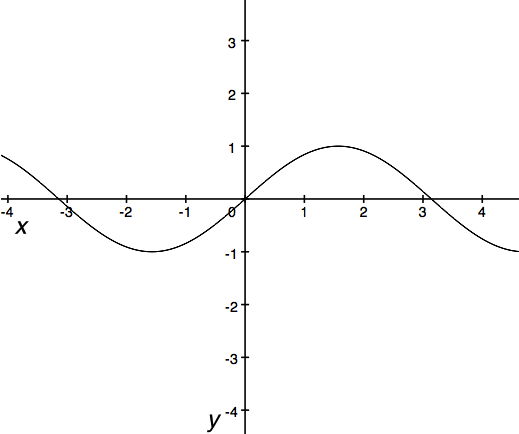#### Question 18 18. If the following graph is f(x), then which graph below could be f'(x)?#### Question 19 19. The function v(t) represents the velocity of an object as a function of time. Given the graph of v(t) below, which of the following statements is true?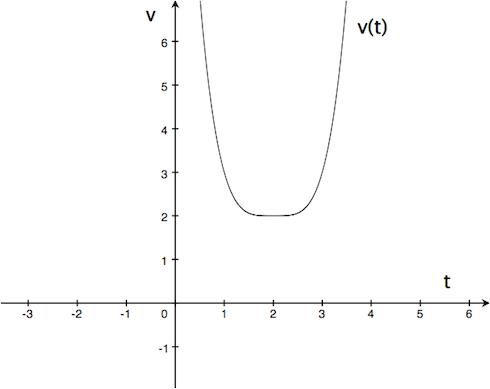#### Question 20 20. The derivative below may have been calculated from which function f(x)?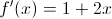### Page 5

#### Question 21 21. What is the derivative of g(t)? Assume C is a constant.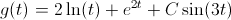#### Question 22 22. Find the second derivative of f(t) shown below.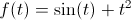#### Question 23 23. Find the derivative df/dx.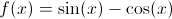#### Question 24 24. If h(t) is the height of an object as a function of time, then which of the following statements are true about h(t)? (Assume t>0.) A) When hitting the ground (at h(t)=0), the object's velocity is -2. B) When hitting the ground (at h(t)=0), the object's acceleration is -2. C) The object hits the ground at t=2.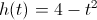#### Question 25 25. Evaluate the derivative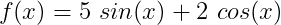#### Calculating Derivatives Chapter Exam Instructions

Choose your answers to the questions and click 'Next' to see the next set of questions. You can skip questions if you would like and come back to them later with the yellow "Go To First Skipped Question" button. When you have completed the practice exam, a green submit button will appear. Click it to see your results. Good luck!

Support